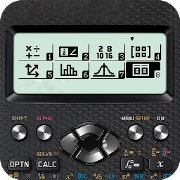# Calculator 570 ex 991 ex - Fraction calculator fxEveryone
7,864
·
Offers in-app purchases
As a user of calculator 991 ex 570ex, I am confident that the 991 EX 570ex is quite possibly the best classroom calculator they have ever produced.

Aimed squarely at the Secondary school, this would be the calculator 991 ex 570ex of choice for any student. The favorite calculator for student. Complete calculator for high school and university student.

Some of the new features:

NUMBER
See answers in textbook style with fractions, brackets, surd (roots), or any combination.
The new Random Integer function makes it easy to simulate
Factorising large numbers into powers of prime factors with a single FACT command.

ALGEBRA
Solve equations and systems of equations or inequalities. The calculator will solve algebraically any polynomial equation up to 4th degree (quartics). It can find a root for any general equation.
Similarly systems of simultaneous equations now go up to four equations in four unknowns. Also, by using the Inequality function on a quadratic, cubic or quartic polynomial.
Full symbolic calculator

MATRICES
Matrices can now be input up to 5 x 5, with the calculator 991 ex 570ex happily finding determinants, sums, products and even inverses.

STATISTICS
Statistical tables - Binomial, Poisson and even Normal distributions are now covered completely. For example, one can calculate the probability that three coins out of five will show Heads; the probability that a person is taller than two standard deviations from the mean; a two-tailed 95% Normal confidence interval. All with a few taps - just entering the values and reading off the result.  Note to CASIO - add in Chi-Squared values and you will have all the lab scientists and social scientists queueing up as well!
- Mean, Sum, Population Standard Deviation, Sample Standard Deviation, Min, Max, Median, Q1, Q3
- Multifunction calculator: algebra, complex, calculus, matrix, vector, ...

REGRESSION
- Linear regression
- Logarithm regression
- Exponential regression
- Power regression
- Inverse regression
- калькулятор для студента

OTHER FUNCTIONS
- Vectors - including Scalar and Cross Product up to 3D vectors
- Calculus - numerical differentiation and integration
- Complex Numbers - Work with both Modulus/Argument and Cartesian form
- Support full trig functions калькулятор sin cos
- Programmable calculator: supports Mathmatica language
- Graphing calculator: Cartesian, polar, parametric, implicit function.
- Unit converter: currency converter, length converter, volume converter, ...
- Math formulas and physic formulas
- Fraction calculator: support fraction, mixed fraction and convert fraction to decimal, easy solve fractions problems
- Complex calculator: supported calculate with complex number, sin cos tan calculate with complex number
- Beautifully scientific calculator: various themes and fonts
- Calculator digits: supported calculate with infinity number of digits.
- Simulate for ti 89 ti-89 ti 84 ti 84+ ti84: supported draw graph, fraction calculating, programming
- Simulate for texas, ti84+ calculator

SUMMARY
Natural display, powerful new functions and the same ease of use in an affordable calculator 991 ex 570ex from one of the world's most trusted calculator manufacturers make this the most highly recommended product for school use currently on the market. Design beautifully scientific, so you can use calculator one hand

KEYBOARD
The calculator include two modes: full scientific calculator and simple scientific calc

научный калькулятор
инженерный калькулятор
калькулятор корень
grafix taschenrechner
Collapse

Review Policy
4.8
7,864 total
5
4
3
2
1

## What's New

# 4.1.0
Show real-valued for n-th root function
Stability and minor improvement
Collapse

Updated
August 8, 2019
Size
15M
Installs
100,000+
Current Version
4.1.1-beta-04-08-2019-00-release
Requires Android
4.1 and up
Content Rating
Everyone
In-app Products
\$1.99 per item
Permissions
Offered By
LisyThien
Developer